# Standard deviation versus standard error

A topic which many students of statistics find difficult is the difference between a standard deviation and a standard error.

The standard deviation is a measure of the variability of a random variable. For example, if we collect some data on incomes from a sample of 100 individuals, the sample standard deviation is an estimate of how much variability there is in incomes between individuals. Let’s suppose the average (mean) income in the sample is $100,000, and the (sample) standard deviation is$10,000. The standard deviation of $10,000 gives us an indication of how much, on average, incomes deviate from the mean of$100,000.

A standard error is a measure of how precise an estimate is. The estimate itself will be of some parameter of interest, such as a mean, or perhaps a regression coefficient. The standard error is the standard deviation of the estimator in repeated samples from the population. As such, the standard error concerns variability in an estimator from sample to sample. Let’s suppose we are interested in estimating the mean income in the population. The sample mean is then equal to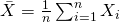To find the standard error of the sample mean, we find its variance, and then take square roots: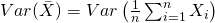If we assume the observations are independent, the variance of the sum is the sum of the variances, and we have: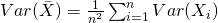If we now write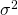for the variance of the variable in the population, we have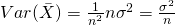The standard error is then just the square root of this quantity, so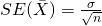This formula shows us that as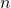, the sample size increases, the standard error decreases. That is, as the sample size gets larger, the standard error gets smaller. This makes sense – the more data we have, the more precise our estimate is.

Also notice that the larger the standard deviation in the population of the underlying variable, the larger the SE. Usually we don’t know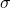, and to estimate the standard error we plug in the sample standard deviation in place of the unknown (true) standard deviation.

Returning to the income example, we had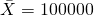, and a sample standard deviation of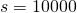. Remembering that the sample size is 100, the estimated standard error of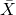is then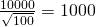If we were to take another sample with larger size, we would expect (on average) to get a smaller SE, but we do not expect the sample standard deviation to be higher or lower (although of course this will vary from sample to sample, due to sampling variability).

### 4 thoughts on “Standard deviation versus standard error”

1.why is the formula sometimes used: (p̂(1-p̂)/n)^1/2?

•2.